1

Prologue.

...Physics is like a cake :
(1)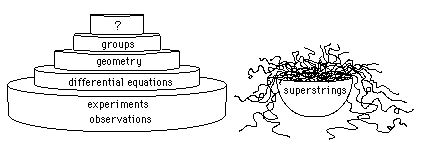- First floor : observations, experiments.
- Second floor : differential equations.

- Third floor : geometry

- Fourh floor : groups theory.

Groups rule geometry, which fathers beautiful differential equations.

With differential equations we build things, which then are used to explain or predict what we call physical facts.

...Historically men began to study and to codify facts, observations, performing measurements. Then they imagined conservations laws, and "physical laws". At the begining of the century they began to think that physical laws could have something to do with geometry.

At the same period, Felix Klein asked :

What is a geometry ?

Notice he said "a geometry" and not "geometry" (Erlangen's program)

...Klein, Lie, Cartan and others showed that something lied under geometrical appearence. Geometry was not the last floor, the nec plus ultra of knowledge in physics. From a group structure one can build a geometry.

In the following we will try to show the link between groups, geometry and physics.
By the way, upon groups, what ?

...I would tend to say : logics. But logics is a flat whose last occupier was Kurt Gdel, a dangerous pyromaniac. With his well-known theorem he put fire to the furniture,which was completely destroyed. Since this tragedy, the room is unoccupied.

...That's for I put a question mark there.

Groups.

...What's a group ? In the following we limit the investigation to dynamic groups of physics : a set of square matrixes (n,n) obeing defined axioms. Theses matrixes g, elements of a group G, act each on another through classical (line-column) matricial multiplication . Among these square matrices we find unity matrixes.
(1-bis)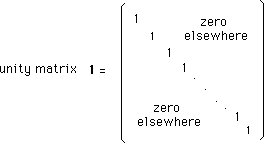...A group obeys the axioms defined by the Norvegian mathematician Sophus Lie. These axioms apply to objects which are much more general than matrixes sets. But we will limit our glance on this peculiar world and use the matrix-multiplication :

x

1 - First axiom of groups'theory :

The product of two elements g1 and g2 of a group G :
(2)

g3 = g1 x g2

obeys :
(3)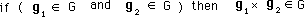Let us give an example of a group of matrix, which depends on a single parameter a . The element is :
(4)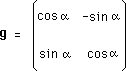The product of two elements gives :
(5)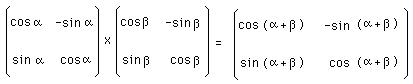or :
(6)

g(a) x g(b) = g( g ) = g( a + b ) = g ( g )

We can write the matrix-product :
(7)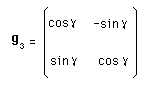which is similar to g1 and g2 , i.e :
(8)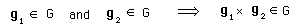Example to the contrary : Consider the following set of matrixes which depend on a single parameter a
(9)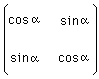The product of two elements gives :
(10)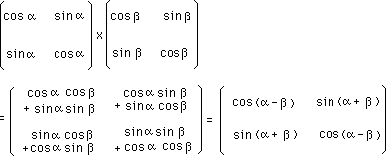which is basically different from (5).

2 - Second axiom of groups'theory :

In the set of elements we must find a peculiar one, called neutral element e , which, combined to any other element, obeys :
(11)

g x e = e x g = g

In groups whose elements are square matrixes this neutral element e is always the unity matrix 1 .
(12)

g x 1 = 1 x g = g

Notice we use lightfaced types to describe scalars and bold types for other objects : square or line or colomn matrixes.

(13)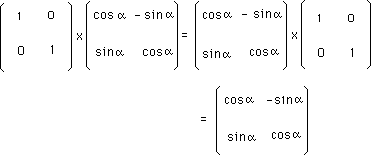Remark that :
(14)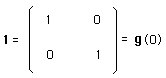Index Dynamic Groups Theory